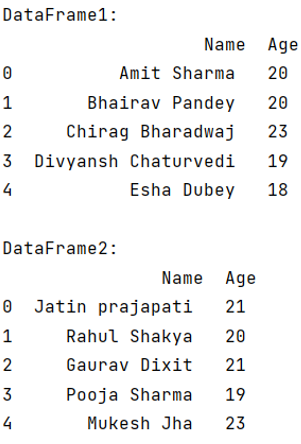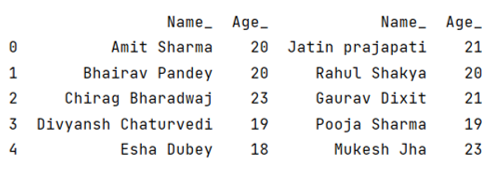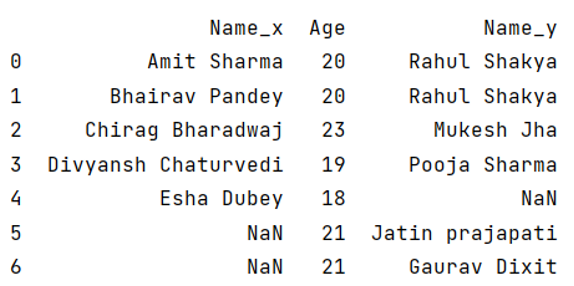# What is the difference between join and merge in Pandas?

Learn about the main differences between join and merge in Python Pandas.
Submitted by Pranit Sharma, on May 23, 2022

Pandas is a special tool which allows us to perform complex manipulations of data effectively and efficiently. Inside pandas, we mostly deal with a dataset in the form of DataFrame. DataFrames are 2-dimensional data structure in pandas. DataFrames consists of rows, columns and the data.

Pandas provides numerous ways to combine two Series or DataFames in order to perform effective and efficient data analytics. Sometimes our required data is not present in a single DataFrame and in that case we need to combine two or more DataFrames.

Pandas merge and pandas join are both the methods of combining or joining two DataFrames but the key difference between merge and join method allows us to combine the DataFrames on the basis of the index i.e., the row value where as merge method allows us to combine the DataFrames on the basis of specific columns instead of index values.

For better understanding, let us first create a DataFrame,

```# Importing pandas package
import pandas as pd

# Creating a Dictionary
dict1 = {
'Name':['Amit Sharma','Bhairav Pandey','Chirag Bharadwaj','Divyansh Chaturvedi','Esha Dubey'],
'Age':[20,20,23,19,18]
}

dict2 = {
'Name':['Jatin Prajapati','Rahul Shakya','Gaurav Dixit','Pooja Sharma','Mukesh Jha'],
'Age':[21,20,21,19,23]
}

# Creating a DataFrame
df1 = pd.DataFrame(dict1)
df2 = pd.DataFrame(dict2)

# Display DataFrame
print("DataFrame1:\n",df1,"\n")
print("DataFrame2:\n",df2,"\n")
```

Output:Now we will apply merge and join separately on these DataFrames to understand the functional difference.

## 1) join() Method

```# Using join method
df_join = df1.join(df2, lsuffix='_')

# Display method
print(df_join)
```

Output:Here, the join method combines the two DataFrames on the basis of their indexes, and we can observe from the above example, that the second DataFrame is simply added to the first DataFrame with properly aligned rows. Also, since our column names are same for both the DataFrames, we have assigned a left suffix to the first DataFrame to distinguish the two DataFrames and to prevent from overlapping.

## 2) merge() Method

```# Using merge method
df_merged = df1.merge(df2, on='Age', how='outer')

# Display result
print(df_merged)
```

Output:What's New (MCQs)

Top Interview Coding Problems/Challenges!

IncludeHelp's Blogs

Languages: » C » C++ » C++ STL » Java » Data Structure » C#.Net » Android » Kotlin » SQL
Web Technologies: » PHP » Python » JavaScript » CSS » Ajax » Node.js » Web programming/HTML
Solved programs: » C » C++ » DS » Java » C#
Aptitude que. & ans.: » C » C++ » Java » DBMS
Interview que. & ans.: » C » Embedded C » Java » SEO » HR
CS Subjects: » CS Basics » O.S. » Networks » DBMS » Embedded Systems » Cloud Computing
» Machine learning » CS Organizations » Linux » DOS
More: » Articles » Puzzles » News/Updates